# Calculating Potential Profit and Loss on OptionsWhen you’re trading options, it’s important to know what’s at stake: What is your maximum gain, maximum loss, and breakeven price on a particular options strategy?  An options profit and loss calculator can help you analyze your trades before you place them.

In this article, we’ll review the Trade & Probability Calculator, which displays theoretical profit and loss levels for options or stock strategies. It helps you determine the likelihood of a strategy reaching certain price levels by a set date, using a normal distribution curve.

## Where do I find it?

The Trade & Probability Calculator is available in the All in One trade ticket on StreetSmart Edge®, circled in red in Figure 1.

Figure 1. StreetSmart Edge dashboard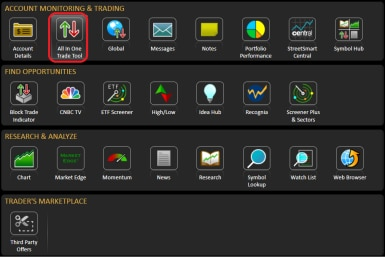Source: StreetSmart Edge

## How does it work?

To get started, you’ll want to select the Trade & Probability Calculator tab (shown in the red box in Figure 2). Then, you’ll want to verify the following graphical default profit and loss (P&L) settings:

• The date of entry (orange line)
• The halfway point (blue line)
• Expiration (purple line)

You can change these defaults by selecting a specific date for any of the three lines. You can also view the numerical probability of reaching a specific target, above and below the current price, by expiration. To do this, move the vertical slider bars with your mouse or enter prices for the lower and upper targets (shown in the yellow box).

Figure 2. Setting P&L dates and target prices to calculate probabilities in the Trade & Probability Calculator tab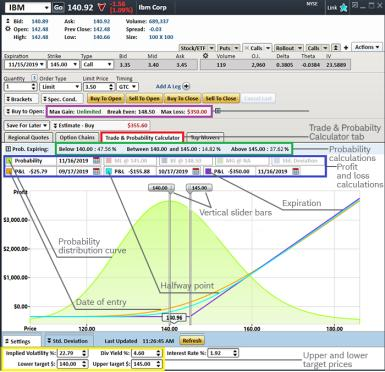Source: StreetSmart Edge

All option pricing inputs can be changed, which allows you to view the price levels and probabilities that are most important to you. For example, you can edit the default implied volatility, dividend yield, and interest rate settings to see how this might affect the outcomes, both numerically and graphically.

Follow this example of how the Trade & Probability Calculator works in action:

Suppose you’re considering the purchase of 1 IBM 11/15/2019 145 Call at a price of \$3.50 when the price of IBM is \$140.92 (see Figure 2). The following price calculations (shown in the purple box) are done automatically:

• Maximum gain (MG) = unlimited
• Maximum loss (ML) = premium paid (3.50 x 100) = \$350
• Breakeven (BE) = strike price + option premium (145 + 3.50) = \$148.50 (assuming held to expiration)

The maximum gain for long calls is theoretically unlimited regardless of the option premium paid, but the maximum loss and breakeven will change relative to the price you pay for the option. These values are also automatically calculated for many other option strategies although the formulas are different.

Now, if you select the Trade & Probability Calculator tab, you’ll see the following additional calculations are done automatically and displayed graphically (shown in the green box in Figure 2):

• Probability of the option expiring below the lower slider bar. If you set the lower slider bar to 140, this would equal 1 minus the approximate Delta of a 140 strike call or (1 – .5244 = .4756 or 47.56%).
• Probability of the option expiring above the upper slider bar. If you set the upper slider bar to 145, this would equal the approximate Delta of the 145 call (.3762) or 37.62%. Since 145 is the call you’re considering for purchase, this is also the same as the probability of the option expiring in the money.
• Probability of the option expiring between the upper and lower slider bar. Assuming the two settings above, this would equal 1 minus the sum of the two previous calculations (1 – (.4756 + .3762) = .1482 or 14.82%).

These probability calculations will change if you alter the lower and upper targets by either moving the slider bars with your mouse or by typing in specific values for the lower target and upper target.

In Figure 2, the profit and loss calculations (shown in the blue box) for the date of entry (orange line), the half-way point (blue line), and expiration (purple line) are estimated, assuming the price of the underlying stock remains unchanged from its current level. In this example, the 145 calls are out of the money initially, so notice how the loss increases as time elapses toward expiration; this is due to time-value erosion

You can click the Refresh button at any time to update the pricing inputs.

To forecast the probabilities of the underlying stock reaching a different price on the various dates displayed, you would place your cursor anywhere on the chart and hold down the left mouse button to create crosshairs that pinpoint the forecasted price and profit and loss amount. As shown in the purple circle in Figure 3, this will calculate the probability of the option reaching that price at any time between now and expiration (“Prob. Touching”) as well as the probability of the option reaching that price level at expiration (“Prob. Expiring”).

Figure 3. Using a specific option price and profit and loss amount to calculate probability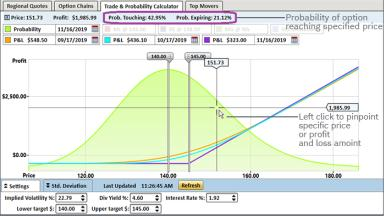Source: StreetSmart Edge

In addition, you can easily make the following calculations, which many option traders find useful (see Figure 4):

• Probability of the option expiring below the upper slider bar. If you set the upper slider bar to 145, it would equal 1 minus the probability of the option expiring above the upper slider bar (1 – .3762 = .6238 or 62.38%). This is the same as the probability of the option expiring worthless.
• Probability of earning a profit at expiration, if you purchase the 145 call option at 3.50. If you set the upper slider bar to the breakeven level of 148.50, this would equal the approximate Delta of a theoretical 148.50 strike call (.2839) or 28.39% (circled in red). Note that while the option was only 4.08 points out of the money when purchased, the stock must increase by 7.58 points for the option to be profitable by expiration. This calculation estimates the approximate probability of that occurring.
• Probability of losing money at expiration, if you purchase the 145 call option at 3.50. Once you have set the upper slider bar to 148.50, this would equal 1 minus the probability of earning a profit at expiration (1 – .2839 = .7161 or 71.61%). As in the previous examples, you’ll note that this is essentially equal to the sum of the other two probability calculations (circled in blue) or 47.56% + 24.06% = 71.62%.

Figure 4. Calculating probability of your options expiring below, at, or above your targets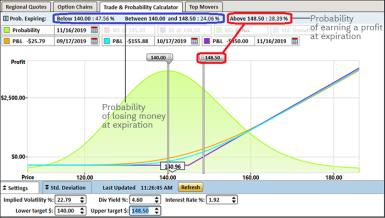Source: StreetSmart Edge

In Figure 5, if you zoom in on the distribution curve, you can choose a closer date for the “Probability” calculation (shown in the red box). This is helpful because it separates the visual profit and loss lines for the various dates. This will also cause the probability calculations (shown in the purple box) to be oriented to the new date you selected rather than the option’s expiration date, which is the default setting.

Figure 5. Calculating the probability using an earlier date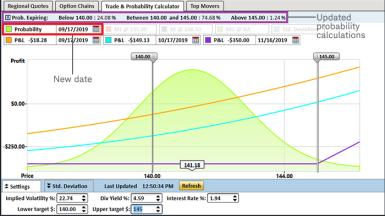Source: StreetSmart Edge

As shown below in Figure 6, you can turn off any of the various visual displays (the normal distribution curve in this example) by simply clicking on the colored box next to the Probability date (circled in red). Likewise, you can turn on a visual line for the ML, BE, MG, or Std. Deviations by clicking on the colored box next to these values (circled in blue).

Figure 6. Customizing the Trade & Probability Calculator display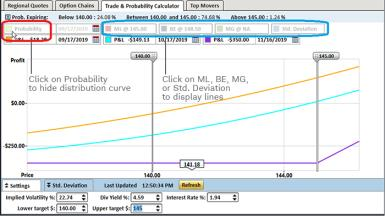Source: StreetSmart Edge

## Keep in mind

While profit and loss calculations assume that option positions will be held until expiration, you can usually close long- or short-option positions prior to expiration by buying or selling them in the market.

• All probability calculations are based on an assumption of stable implied volatility values. Changes in implied volatility could dramatically affect forecasts.
• Delta is often used as an instantaneous forecast of the approximate probability of an option contract expiring in the money. Just keep in mind that Delta is calculated continuously, so it will generally increase or decrease as the underlying stock price changes.
• The projections and forecasts generated by the Trade & Probability Calculator are hypothetical in nature and should not be regarded as indicative of actual investment results.
• Trading and investment decisions should not be based solely upon values or calculations generated by the Trade & Probability Calculator.